Setting up a new grading system should be done in the master space, not the delivery space. These changes can only be implemented by conveners, block conveners, and block key academics.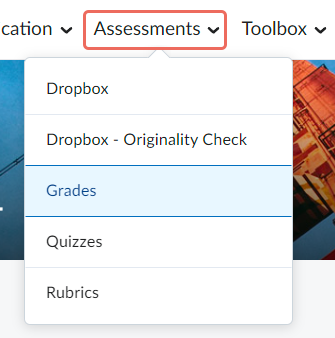2. Select Setup Wizard.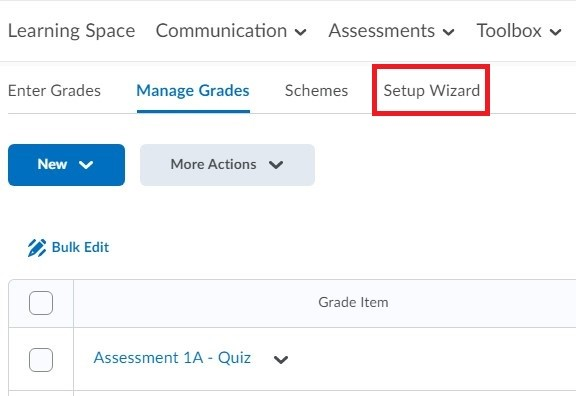3. From here you can view all settings in the current gradebook, including:

• (b) Final Grade to Release
• (e) Manage View Display Options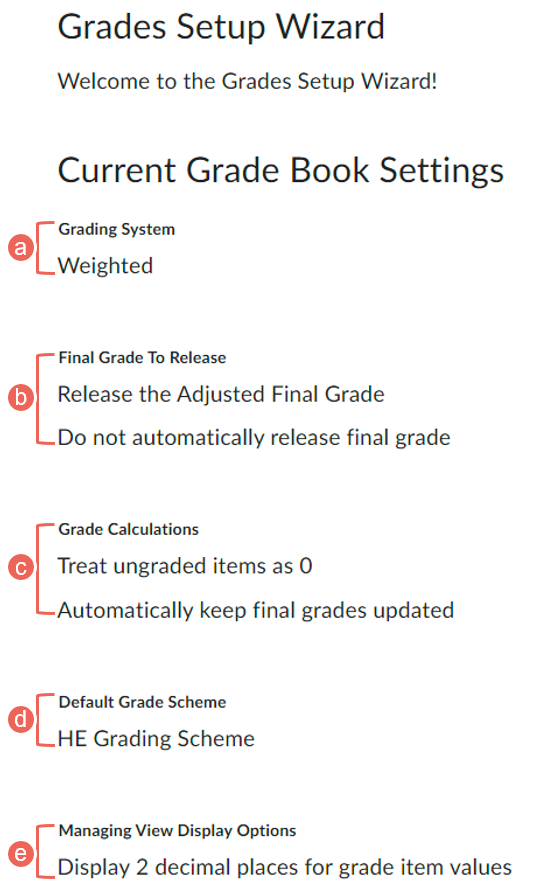## Option One: Weighted System

Under the 'Weighted' system, assessment items are scored out of percentages %. The Final Calculated Grade then becomes the total number of percentages of all the items.

Ensure the Final Calculated Grade always sums to 100% if your units are in Block Mode.

##### (a) Category Weight

If you group assessment items under a Grade Category, all grade items in the category should combine to a weight of 100% of that category, not of the final grade.

For example below in blue highlight, Assessment 4 - Category weight 30%

• Group Submissions - 50%
• Peer Review One - 25%
• Peer Review Two - 25%

Each item weight of the category adds to 100% (50 + 25 + 25 = 100%), so 100% of Assessment 4 - Category (30%) goes towards the final grade.

##### (b) Final Calculated Grade Weight

The Final Calculated Grade displays the total percentages % of each assessment item calculated from the Weight column and should total 100.

For example,

• Assessment 1 - 20%
• Assessment 2 - 25%
• Assessment 3 - 25%
• Assessment 4 - 30%

in total this unit gradebook has 20 + 25 + 25 + 30 = 100%.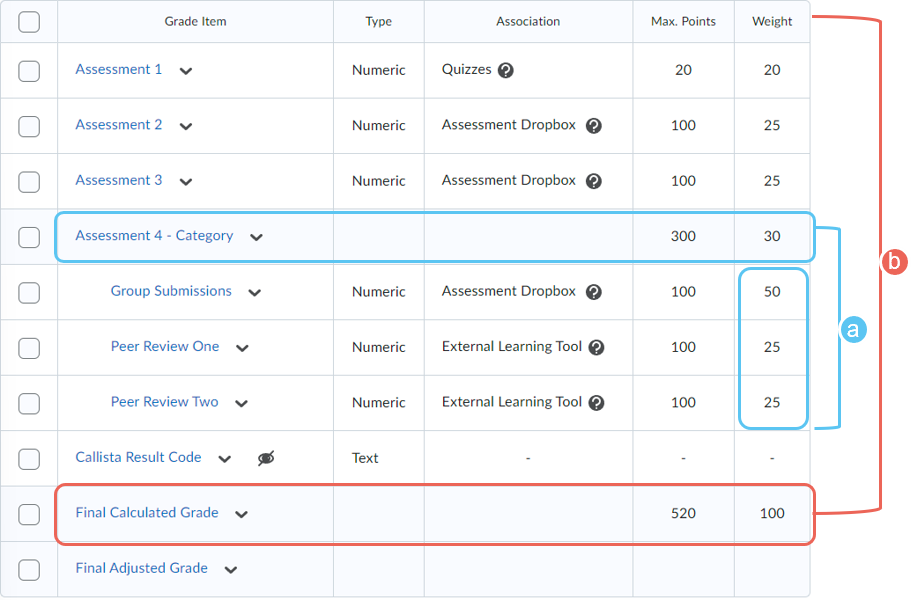If Final Calculated Grade do not add up to 100%, you receive a warning message. Since this will cause issues with the grades return and student final grades, please review each assessment item to ensure they add up to 100%.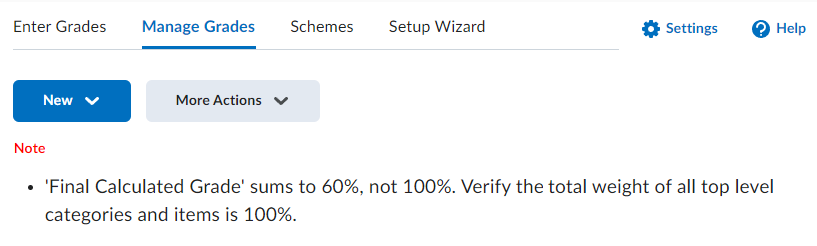For the Weighted gradebook, the Final Calculated Grade column showcases the Total Weight a student has received / Total Weight of the unit.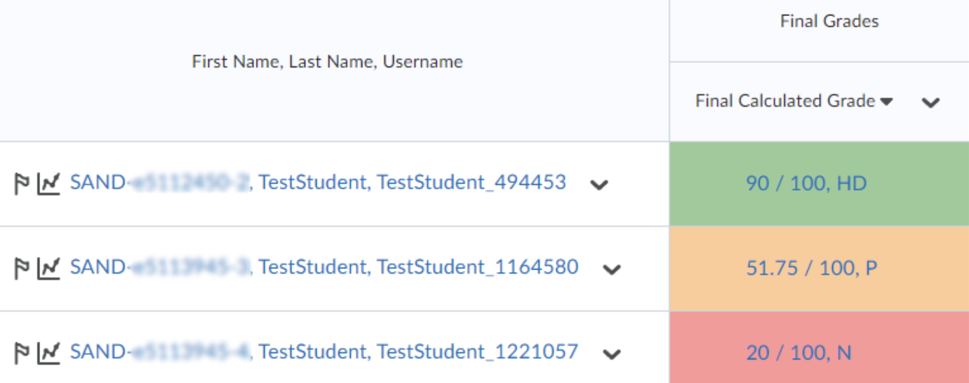##### (c) Max. Points column in Weighted system

Within the Weighted gradebook, you will have both Max. Points and Weight column:

1. Max points: The number of points that each assessment is marked out of, and should also match the Out of Score if associated with an Assessment Dropbox, Rubric, and/or Quiz.
2. Weight: The total weight of each assessment item is calculated from this column and should total 100.

In this example, Assessment 3 is out of 100 points (a) and contributes 25% (b) to the final grades.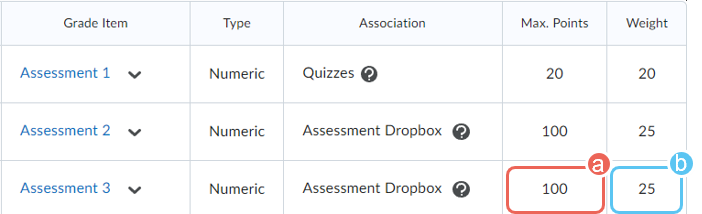## Option Two: Points System

Under the 'Points' system, assessment items are scored out of points. The Final Calculated Grade then becomes the total number of points of all the items.

Recommended for HE Placement units and units that have Pass / Fail assessments.

##### (a) Category in the Points system

For the Points system, the Grade Category will be the sum of all grade items under it.

For example below in blue highlight, Assessment 3 has 3 x Workbook grade items. Each item max points add to the Assessment 3 category (20 + 30 + 50 = 100 Points).

##### (b) Final Calculated Grade in the Points system

The Final Calculated Grade displays the total points of each assessment item calculated from the Max Points column.

Example in red highlight,

• Assessment 1 - out of 60 points
• Assessment 2 - out of 80 points
• Assessment 3 - out of 100 points

in total this unit gradebook has 60 + 80 +100 = 240 Points.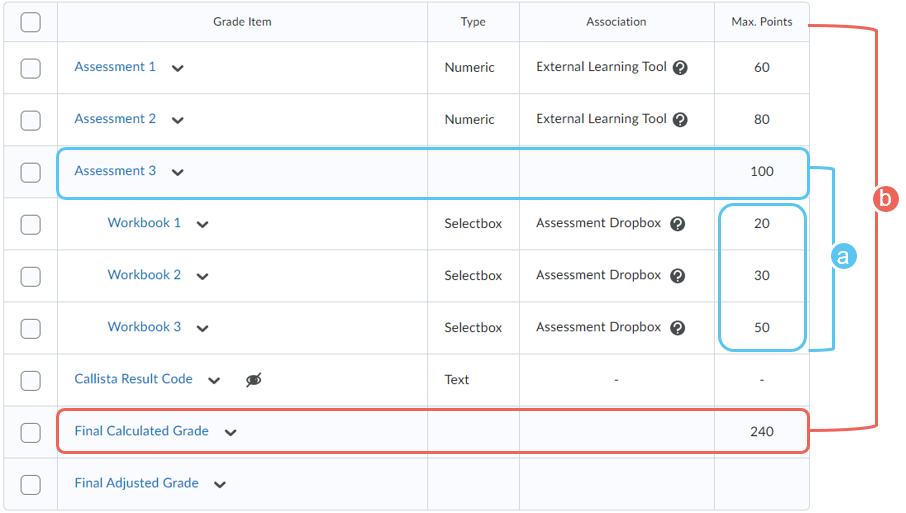For the Points gradebook, the Final Calculated Grade column showcases the Total Points a student has received / Total Points of the unit.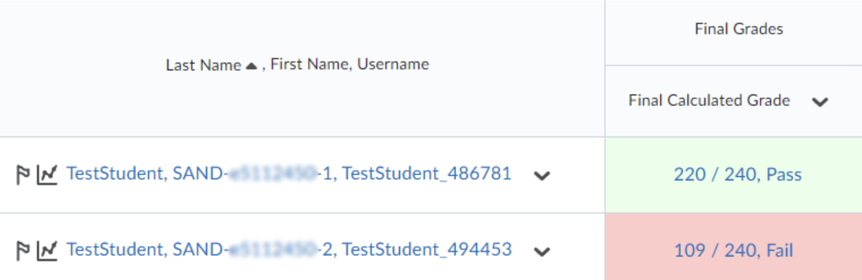## Option Three: Formula System

Under the 'Formula' system, assessment items are scored out of points, similar to the Points system. However, the Final Calculated Grade will act as a custom-defined formula that determines how all assessment items contribute to the calculated final grade.

Use the Formula system when want to calculate final grades using a custom formula & conditions.

For example, you could require that students receive at least 50 points on their first assessment and 60 points on the final assessment to pass a unit.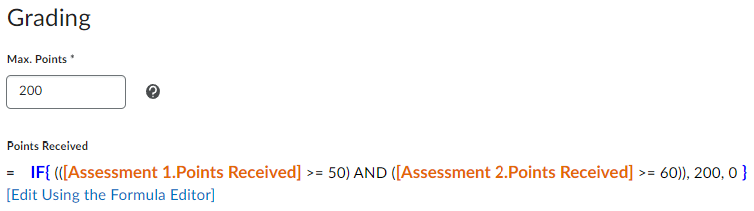This will determine if the students will pass the unit or not based on their received scores.

• Student 1: scored 50 on assessment 1 and 60 on assessment 2 => Pass (in green colour)
• Student 3: scored 40 on assessment 1 and 50 on assessment 2 => Fail (in red colour)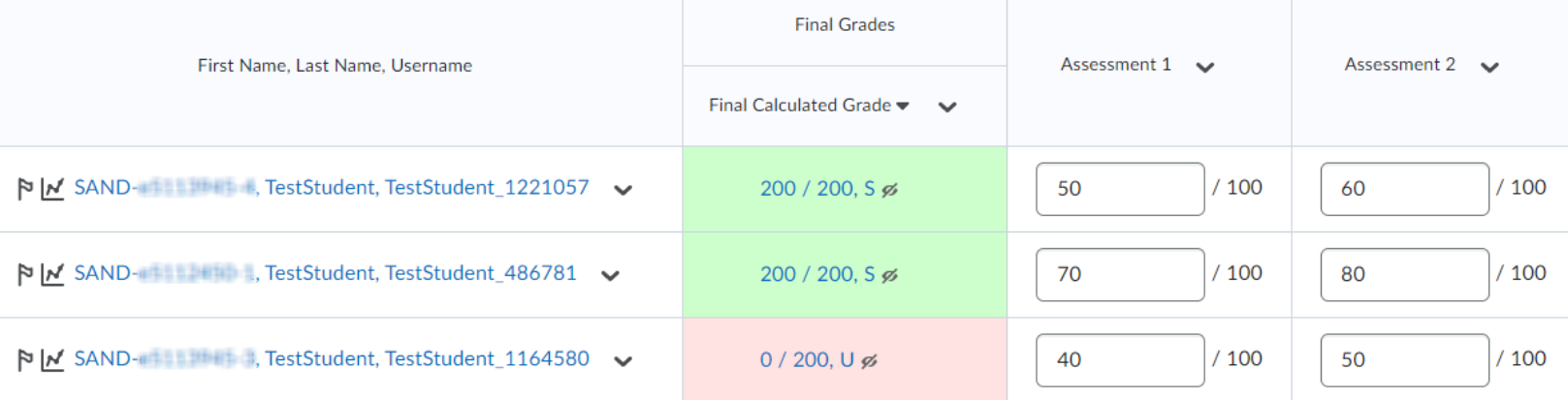Further Support

Contact us This email address is being protected from spambots. You need JavaScript enabled to view it., for information and resources on technology-enhanced learning, or visit VUCollaborateHelp.vu.edu.au.
Contact ITS Service Desk on (03) 9919 2777 or via self-service servicedesk.vu.edu.au for technical support.